Reach Us+44 1704 335730
Particle Swarm Optimization Approach for Estimation of Energy Demand of Turkey | OMICS International
Global Journal of Technology and Optimization
All submissions of the EM system will be redirected to Online Manuscript Submission System. Authors are requested to submit articles directly to Online Manuscript Submission System of respective journal.

# Particle Swarm Optimization Approach for Estimation of Energy Demand of Turkey

Turan Paksoy1*, Eren Özceylan1, NimetYapıcı Pehlivan2 and Gerhard-Wilhelm Weber3

1Selçuk University, Department of Industrial Engineering, Campus, 42031, Konya, Turkey

2Selçuk University, Department of Statistics, Campus, 42031, Konya, Turkey

3Middle East Technical University, Institute of Applied Mathematics, 06531, Ankara, Turkey

*Corresponding Author:
Turan Paksoy
Selçuk University, Department of Industrial Engineering, Campus, 42031, Konya, Turkey
E-mail: [email protected]

Visit for more related articles at Global Journal of Technology and Optimization

#### Abstract

This paper presents an application of Particle Swarm Optimization (PSO) technique to estimate energy demandof Turkey, based on economic indicators.The ec onomic indicators that are used during the model development are: gross national product (GNP), population, import and export figures of Turkey. Energy demand and other economic indicators in Turkey from 1979 to 2005 are considered as the case of this study. The energy estimation model based on PSO (EEPSO) is developed in two forms (linear (EEPSOL) and quadratic (EEPS OQ))and applied to forecast energy demand in Turkey. PSOQ form provided better-fit solution due to fluctuations of the economic indicators. In order to show the accuracy of the algorithm, some comparisons are made with previous studies which are using Ant Colony Optimization (ACO) and PSO. The future energy demand is calculated under different scenarios. The relative estimation errors of the proposed models are the lowe st when they are compared with the Ministry of Energy and Natural Resources (MENR) projection.

#### Keywords

Particle Swarm Optimization, E EPSOL, EEPSOQ, Energy Demand, Forecast, Relative Error, Turkey.

#### Introduction

It is widely known that energy consumption a nd demand level is directly related to the level of development of a country like Turkey. Hence, carrying an idea about energy demand and policy is a matter of serious importance. Turkey, which is a Eurasian country that stretches across the Anatolian peninsula in western Asia and Thrace in the Balkan region of so utheastern Europe, has been one of the fastest growing power m arkets in the world with its young and growing population, rapid urbanization, strong economic growth and low per- capita electricity consumption for two decades (, ).

Turkey’s energy demand has grown rapidly almost every year and will continue to grow along with its economy.The primaryenergy need of Turkey has been gro wing by some 6% perannum for decades. Recent forecasts indic ate that this trend will continue as a result of ra pid urbanization andindustrialization .Turkey’s primary energy sources are hardcoal, lignite, hydropower, oil, natural gas, geothermal andsolar energy, wood, as well as animal and plant wastes. However, the level of energyproduction in T urkey is very low (Figure 1).At present, around 2 6% of the total energy demand is being met by domestic energy sources, while the rest originates from a diversified import-portf olio .

Figure 1: The share of each fuel in total primary energy production and consumption in 2007  (MTOE: million tons of oil equivalents)

Turkey has various sources for primary energy production and consumption (Figure 1). Coal, natural gas and oil consumptions are very close and have 91% in total primary energy consumption, while their production is 63.6% in total primary energy production.In other w ords, only a small percentage of total primary consumption was provided by domestic production. It is expected that by the year 2020, domestic energy consumption will reach 222 M TOE, while domestic production will be at 70 MTOE, or 30% of national demand . These indicators show that Turkey is forced to increase its dependence on foreign energy supplies. Thus, the accurate estimating of energy demand is very critic al factor in the Turkey's energy policy making. The goal of this study is to provide that accurate estimating model of energy dem and using PSO.

In the following section, a bri ef description of the problem and literature survey about the solution is given. In the Section 3, the concept of swarm intelligence and the basic PSO algorithm is

In the following section, a bri ef description of the problem and literature survey about the solution is given. In the Section 3, the concept of swarm intelligence and the basic PSO algorithm is given. Energy demand forecasting model (EEPSO), which is developed for Turkey case, is explained in the Section 4. Results of energy demand forecasting obtained by proposed approach, comparisons with other methods and future projections are presented in Section 5. Finally, the study is concluded in Section 6 with suggestions on future researches.

#### Literature Review

Energy modeling is a subject of widespread current interest among engineers andscientists concerned with problems of energy production and consumption . First applications on energy demand forecasting in Turkey are done by State Planning Organization (SPO). SPO initiated the use of simple regression techniques for energy forecasting at that time . These forecasts consistently predicted much higher values than the consumption that actually occurred.

Modern econometric techniques have been applied for energy planning and estimation of future energy demands in 1984 first (Figure 2). Model for analysis of energy demand (MAED) which is a kind of simulation model and developed by International Atomic Energy Agency (IAEA) was started to be used by Ministry of Energy and Natural Resources of Turkey (MENR) . MAED is used to estimate the medium and long term energy demand, considering the relationships between several factors that affect the social, economic and technologic system of the country .

Figure 2: Important MAED applications and some projections by SPO and realization in Turkey between the 1950-2010 years

Except aforementioned methods, many models have been developed from many researches using various forms of mathematical formulations, which are directly or indirectly related to energy development models to find a relation between energy consumption and income (,, ,,, and). For energy forecasting, statistical models are also considered by Ediger and Tatlıdil,Sarak and Satman, Yumurtacıand Asmaz,Görücü and Gümrah, Aras and Aras , Ediger and Akar andErdoğdu. Except mentioned statistical models, Çelikta and Koçarused Delphi method for evaluating Turkey’s renewable energy future. A two-round Delphi research study was undertaken to determine and measure the expectations of the sector representatives regarding the foresight of renewable energies. First and second round of Delphi study were carried out by using online surveys. Akay and Atak  proposed an approach using gray prediction with rolling mechanism (GPRM) to predict the Turkey’s total and industrial electricity consumption. Ceylan et al.  proposed a new method for estimating transport energy demand using a harmony search approach.

In the energy estimation literature,meta-heuristic methods, which are used to solve combinatorialoptimization problem, have been rarely applied toestimate energy consumption .A summary of techniques, used so far for energy demand forecasting is given in Table 1. In 2004, genetic algorithm model approach began to be used for estimating energy, electricity, transport energy, gas, oil, and exergy demand/consumption byCanyurt et al. , Ceylan and Öztürk , Öztürk et al. , Öztürk et al. , Öztürk et al. , Ceylan et al. , Haldenbilen and Ceylan ,Canyurt and Öztürk , Canyurt and Öztürk. After GA applications, Artificial Neural Networks, that is one of the artificial intelligence techniques, is used for forecasting energy, electricity, transport energy and gas demand/consumption by Görücü et al. , Sözen et al. , Murat and Ceylan , Sözen and Arcaklıoğlu , Hamzaçebi , Sözen , Kavaklıoğlu et al.  and Kankal et al. . Swarm intelligence approach is also applied for forecasting energy and electricity demand of Turkey. While Ant Colony Optimization(ACO) is used by Toksarı , ; PSO is used by Ünler  to estimate Turkey energy demand.

In addition to studies mentioned above, proposed approach (EEPSO) is applied to estimate energy demand of Turkey in this study. The proposed model is compared ACO  and PSO  models to show the advantages and availability of proposed method.

#### Particle Swarm Optimization

The Particle Swarm Optimization is one of the recent meta-heuristic techniques proposed by Kennedy and Eberhart  based on natural flocking and swarming behavior of birds and insects.It isinitialized with a population of random solutions and searches for optima by updating generations. In PSO, the potentialsolutions, or particles, move through the problem space by following the current optimum particles . The concept of PSO gained in popularity due to its simplicity. Like other swarm-based techniques, PSO consists of a number of individual refining their knowledge of the given search space . However, unlike GA, the PSO algorithm has no evolutionary operators, such as crossover and mutation. The individuals in a PSO have a position and a velocity and are denoted as particles. The PSO algorithm works by attracting the particles to search space positions of high fitness. Each particle has a memory function, and adjusts its trajectory according to two pieces of information, the best position that it has so far visited, and the global best position attained by the whole swarm .

The system is initialized with a population of random solutions (particles) and searches iteratively through the d-dimensional problem space for optima by updating generations .Each particle keeps a memory of its previous best position, pbest, and a velocity along each dimension, represented as Vi= (νi1, νi1,….,νid).When a particle takes all the population as itstopological neighbors, the best value is a global best and is called gbest.Many attempts were made to improve the performance of theoriginal PSO algorithm and several new parameters were introducedsuch as the inertia weight. The canonical PSO withinertia weight has become very popular and widely used in manyscience and engineering problems .

The PSO concept consists of, at each time step, changingthe velocity (V) of (accelerating) each particle toward itspbestlocation according to Eq. (1). The newposition of the particle is determined by the sum ofprevious position and the new velocity which is given inEq. (2) :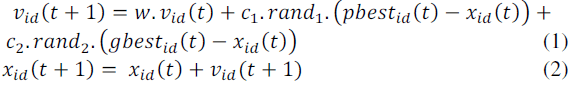Where c1 and c2 determine the relative influence of the social and cognitive components (learning factors), while rand1 and rand2 denote two random numbers uniformly distributed in the interval [0,1].w is a parameter called inertia weight used to control the impact of the previous velocities on the current one. In proposed PSO, inertia value of the equation changes on the each iteration. This change is based on the logic of decreasing from the value determined to minimum value according to inertia function. The objective is to converge the created speed by diminishing on the further iterations; hence more similar results can be obtained . Inertia function is obtained as follow: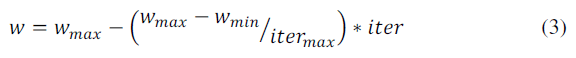#### PSO Energy Demand Estimation (EEPSO)

Four indicators (population, GDP, import and export) were used in energy demand estimating models which are proposed based on PSO. These indicators are commonly used in literature (, ,  and ) and believed that energy demand of a country is mostly affected by them. Table 2 shows four indicators and energy demand of Turkey between 1970 and 2005. The data are collected from Turkish StatisticalInstitute (TSI)  and the MENR. Data until 2005 is used to make a comparison other models which are developed for the same problem.

As it seen in Table 2, it is clear that there is a linear relationship between four indicators and energy demand. For example, while GDP, population, import and export of Turkey increased 3.4; 0.63; 22 and 31.5 times respectively, energy consumption of Turkey has increased 1.98 times between 1979-2005 years.

In this study, the estimation of energy demand based on economic indicators was modeled by using various forms, e.g. linear (Eq. (4)) and quadratic (Eq. (5)). Linear form (EEPSOL) can be expressed as,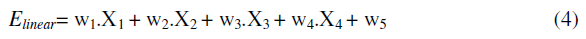and quadratic form (EEPSOQ) can be expressed as,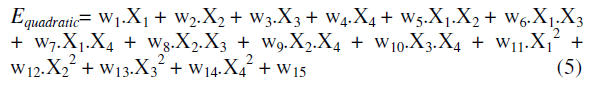EEPSO model optimizes coefficients (wi) of the design parameters(Xi), which are included by models, concurrently.In energy demand estimating, the aim is to find the fittest model to the data. The fitness function of the model is given by,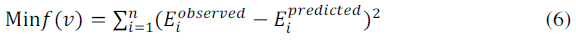where Eobserved and Epredictedare the actual and predicted energy demand, respectively, n is the number of observations. The EEPSO algorithm is composed of 4 mainsteps: Step1. Initialize a defined population of particles with random positions (Xi), velocities (Vi) and set iteration number, c1, c2 and wmax-min values. For the problem, random positions are input variables values.

Step2.Compute the objective valuesof all particles. Define own best position of each particle and its objective value pbest equal to its initial position and objective value, and define global best position and its objective value gbest equal to the best initial particle position and its objective value. For the problem, objective function is total forecasting error which uses sum square errors.

Step3.Change velocities and positions by using Eqs. (1) and (2) according to obtained feedbacks from pbest and gbest.

Step4. Repeat step 2 and step 3 until the predefined number of iterations is completed. For the proposed model, iteration number is used for the stopping algorithm.

#### Estimation of Turkey Energy Demand

Turkey’s energy demand by using the structure of the Turkey socio-economicconditions is the main objective of this study. EEPSOmodels (linear (EEPSOL) and quadratic (EEPSOQ)) are developed to estimate the future energy demand values based on population,GDP (gross domestic product), import and export figures (Table 2).The EEPSO model was coded with MATLAB 2009 and run on a Pentium IV, 1.66 GHz, 2 GB RAM notebook computer. One of the important problems is setting the best parameters of PSO. Four important factors, particle size, inertia weight (w), maximum iteration number (iter) and c1,2are considered. According to Shi and Eberhart  c1 and c2 have a fixed value as 2. Hence, in this study these fixed values are also used.The other parameters except inertia weight (w) is considered with the same of Ünler ; as particle size: 20 and asmaximum iteration number: 1000. A few statistical experiments are performed in order to find the best value of wmax and wmin. As a result of the statistical analysis, wmax and wmin are determined as 0.7 and 0.5. Twenty-seven data (1979–2005) were used to determine the weighting parameters of EEPSO models. EEPSOL and EEPSOQ models with aforementioned parameters and data were tested 20 times and best results were considered.Following EEPSO (linear and quadratic) equations have been obtained for energy forecasting. In the linear form, coefficients obtained are givenbelow: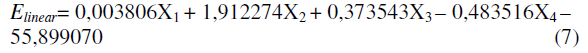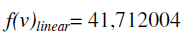In the quadratic form of the proposed EEPSO model, coefficients obtained are given below: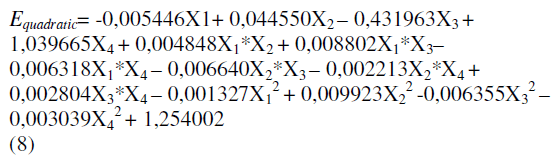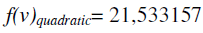where X1 is GDP, X2 is population, X3 is import, X4 is export and f(v) is sum of squared errors. Ten data (1996–2005) were used to validate the models. Table 3 shows relative errors between estimated and observed data.

According to Table 3, proposed EEPSO approach for energy demandestimation are very robust and successful. Although the largest deviation is 3.37% for linear form and -2.38% for quadratic form, they are quite acceptable levels. The largest deviations are obtained in 1999 because of the decreasing in GDP, import and export in that year (Table 2). Results show that quadratic form provided better fit estimation than the linear form due to the fluctuations of the economic indicators.It is also observed that while proposed EEPSOL approach is providing better fit estimation than Toksarı  and Ünler  in linear form (Figure 3), EEPSOQ remains between Toksarı  and Ünler  in quadratic form (Figure 4). When twenty seven data is considered (1979-2005), proposed approach finds less relative error than the other studies in both of linear and quadratic forms. Tables 4 and 5 give coefficients and forecasting relative errors of each study in linear and quadratic forms.

In order to show the accuracy of proposed models, three scenarios are used for forecasting Turkey’s energy demand in the years 2006–2025 and they are compared with Toksari’s  ACO, Ünler’s  PSO models and MENR projections. Each scenario is explained below ;

Scenario 1: It is assumed that the average growth rate of GDP is 6%, population growth rate is 0.17%, import growth rate is 4.5%, and export growth rate is 2% during the period of 2006–2025.

Scenario 2: It is assumed that the average growth rate of GDP is 5%, population growth rate is 0.15%, import growth rate is 5%, and proportion of import covered by export is 45% during the period of 2006–2025.

Scenario 3: It is assumed that the average growth rate of GDP is 4%, population growth rate is 0.18%, import growth rate is 4.5%, and export growth rate 3.5% during the period of 2006–2025.

Table 6, Figures 5 and 6 show the estimated values for two forms of proposed approach for the Scenario 1. Proposed EEPSOQ form gives lower forecasts of the energy demand than the Toksarı , Ünler  and MENR projections (Figure 6). The proposed EEPSOL form also gives lower estimates of the energy demand than the Toksarı  and MENR projections. It gives a bit higher estimation values than Ünler’s  linear model (Figure 5). As a result, proposed quadratic form is better than proposed linear form according to Scenario 1.

Figure 5: Future projections of total energy demand in MTOE according to Scenario 1 (linear form)

Figure 6: Future projections of total energy demand in MTOE according to Scenario 1 (quadratic form)

Table 7, Figures 7 and 8 show the estimated values for two forms of proposed approach for the Scenario 2.As can be seen from Figure 7, three linear studies (Toksarı ; Ünler ; EEPSOL) give nearly the same estimation that proposed EEPSOL method is lower than Toksarı  higher than Ünler . ProposedEEPSOQ form gives lower forecasts of the energy demand thanToksarı  andÜnler  (Figure 8).As a result, proposed quadratic form is better than proposed linear form according to Scenario 2, too.

Figure 7: Future projections of total energy demand in MTOE according to Scenario 2 (linear form)

Figure 8: Future projections of total energy demand in MTOE according to Scenario 2 (quadratic form)

Estimated values for two forms of proposed approach for the Scenario 3 could be seen in Table 8 and Figures 9 and 10. When Figure 9 presents that the estimated values for linear form of proposed method (EEPSOL), EEPSOL gives lower estimates of energy demand than Toksarı’s  linear model and MENR projections. It is also lower than Ünler’s  linear model until 2011 then they give nearly the same estimation. In quadratic form, as it can be seen from Figure 10, proposed EEPSOQ model gives the lowest forecasts of the energy demand. As it is in Scenario 1 and 2, proposed quadratic form is better than proposed linear form according to Scenario 3.

Figure 9: Future projections of total energy demand in MTOE according to Scenario 3 (linear form)

When all three scenarios are examined, proposed EEPSO method with its linear and quadratic forms gives lower and realistic estimations than MENR projections. Developed EEPSOL model always estimate lower values than Toksarı’s  linear model and also estimated energy demand values of EEPSOL are closer to Ünler’s  linear model. When two forms are considered, it is clear that EEPSOL gives lower forecasts of the energy demand that the EEPSOQ. Hence, both EEPSOL and EEPSOQ should be used to estimate the energy demand of Turkey to make more efficient and realistic estimations.

#### Conclusion

Planning and estimating of energy is quite important to make sustainable energy policy for countries. The relation between energy demand and socio-economic development of a country shows the importance of theneed for systematic optimization of the energy demand estimation inTurkey. That’s why, in this study, estimation of Turkey’s energy demand based on PSO is suggested via considering GDP, population, import and export indicators. Two forms (linear and quadratic) of the EEPSO model are developed because of fluctuations of the economic indicators. 27 years data (1979-2005) is used to show the availability and advantages of proposed approach than the previous studies. Three scenarios are proposed to forecast Turkey’s energy demand in the years 2006–2025 using the two forms of the EEPSO. They are compared with the MENR, Toksarı’s  and Ünler’s  projections. In this study, the following main conclusions may be drawn:

• While the largest deviation is 3.37% for linear form (EEPSOL), the largest deviation is 2.38% for quadratic form (EEPSOQ) in modeling with 27 years data (1979- 2005).Then, it is observed that quadratic EEPSO provided better fit solution than linear formdue to the fluctuationsof the economic indicators.

• According to results of modeling and scenario analysis, it is clear that particle swarm optimization technique gives better forecasts than ant colony optimization technique.

• While EEPSOL gives lower relative error than Toksarı’s  linear model with 8.77% and Ünler’s  linear model with 2.12%, EEPSOQ gives lower relative error than Toksarı’s  quadratic model with 22.95% and Ünler’s  quadratic model with 22.16%.

• The estimation of energy demand of Turkey using EEPSOQ form is underestimated and EEPSOL form has close estimations when the results are compared with Toksarı’s , Ünler’s  and MENR projections (2006-2025). So, it can be say that EEPSO forms, especially EEPSOQ is more realistic and acceptable.

• All test problems and scenarios show that both of linear and quadratic forms should be reliable.

It isconcluded that the suggested models are satisfactory tools forsuccessful energy demand forecasting. The results presented hereprovide helpful insight into energy system modeling. They could be alsoinstrumental to scholars and policy makers as a potential tool fordeveloping energy plans.

Future works should be focused on comparing the methods presentedhere with other available tools. Forecasting of energy demand can also be investigated with bee colony optimization, artificial bee colony, bacterial foraging optimization, fuzzy logic, artificial neural networks or other meta-heuristic such astabu search, simulated annealing, etc. The results of the differentmethods can be compared with the PSO methods.

#### References

Select your language of interest to view the total content in your interested language

### Article Usage

• Total views: 12486
• [From(publication date):
June-2012 - Nov 15, 2019]
• Breakdown by view type
• HTML page views : 8639Can't read the image? click here to refresh